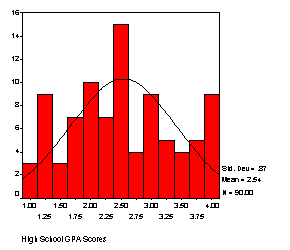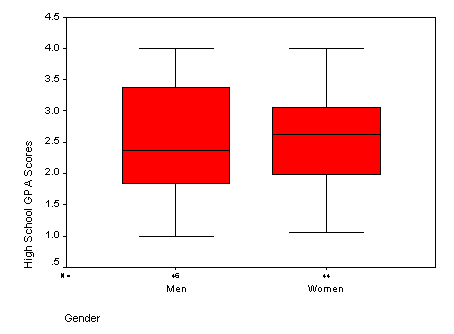Examples of Distributions and Descriptive Graphs

Frequency Histogram

Discrete values of categories are used on the horizontal (x) axis, and the number of scores that fall into that category (i.e., frequency) appears on the vertical (y) axis.  A normal curve is overlaid so that one can easily see how it departs from normal.  This distribution looks close to normal, although there is a pretty high peak in the middle and a some high frequencies in the right and left tails.Box Plots

Below are box plots or sometimes called “box and whiskers.”   The center line is the median, the top and bottom of the box is the upper and lower “hinge” (approximately the upper and lower quartiles), and the endpoints of the whiskers represent the approximate range.  SPSS will sometimes print “outliers” and “extreme points” (denoted by circles and asterisks) that lay outside the end of the whiskers, in which case the whiskers are not really the lowest and highest scores.  Defining an outliers and extreme points is rather subjective. SPSS uses z-scores greater than a certain value to define these.  However, identifying outlying points is probably best left to the researcher to define—you may want to consider some points outliers even though SPSS does not or SPSS may identify something as extreme that you do not.  Box plots are probably the most useful when you are comparing two groups.Stem and Leaf

The Stem and Leaf combines a histogram with actual data points. If you imagine turning the figure on its side, it resembles a frequency histogram.  The “stems” are categories for the scores, usually identified by the first digit of the score, and the “leaves” are the last digit of the score.  Often, some type of rounding is used, depending on whether there are decimals or the number of digits is large.  The intention is to allow you to identify exact scores in the figure and also get a sense of the shape of the distribution.

High School GPA Scores Stem-and-Leaf Plot for

GENDER= Men

Frequency    Stem &  Leaf

6.00        1 .  011123

8.00        1 .  56778889

9.00        2 .  000112222

9.00        2 .  555556668

3.00        3 .  023

5.00        3 .  55788

6.00        4 .  000000

Stem width:      1.00

Each leaf:       1 case(s)

High School GPA Scores Stem-and-Leaf Plot for

GENDER= Women

Frequency    Stem &  Leaf

8.00        1 .  01233334

3.00        1 .  699

6.00        2 .  000134

13.00        2 .  5556666779999

8.00        3 .  00011112

4.00        3 .  5789

2.00        4 .  00

Stem width:      1.00

Each leaf:       1 case(s)